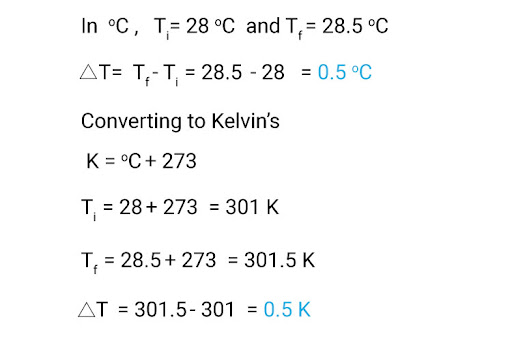# If, Rate of thermal enerfy transfer = 2.7kwVolume of a water tank = 4.5m^3Water temperature = 28°CDensity of water =  1000kgm-3 & c=4200Jkg-1K-1What will be the rise in water temperature the heater can produce in 1 hour?

A: When it comes to solving numerical questions, it really helps to first write out the main equation, and see what quantities do we need: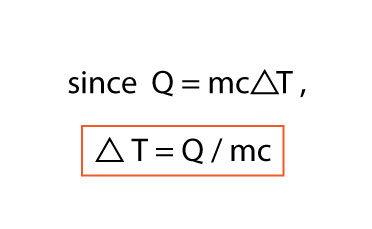We can now see that we need the following:
• Mass (m)
• Specific heat capacity (c)
• Amount of heat energy absorbed (Q)
To find the mass of water that absorbed the heat energy Q, we use density to our advantage: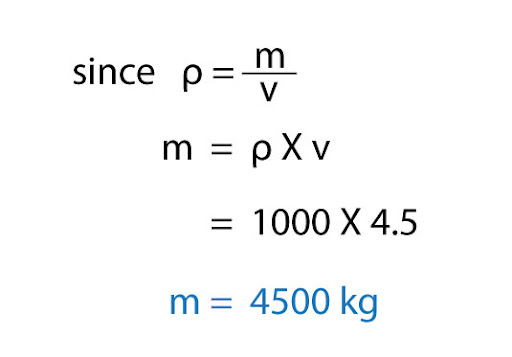Specific heat capacity is already given, i.e. c = 4200 Jkg-1K-1. Finally, how do we find the amount of heat energy absorbed (Q)? For that, we’ll actually use the formula for power to our advantage: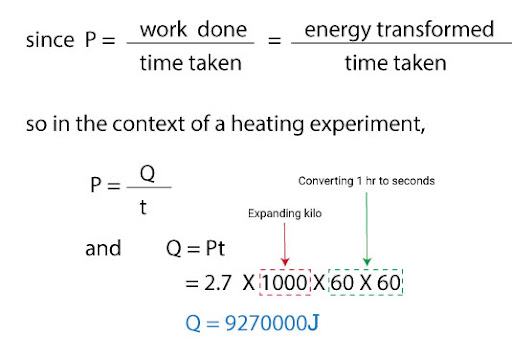Now that we have everything we need, all we have to do is plug it in the main equation!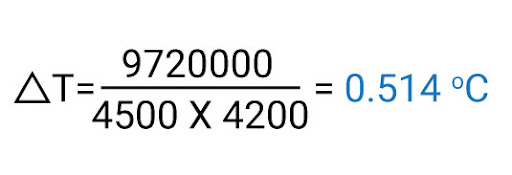This means that the temperature of the sample of water will increase by 0.514 deg C, so the final temperature will be 28.514 deg C. You may have noticed how the units for specific heat capacity contain the unit for Kelvin K - so how could my final answer be written in Celcius? That’s because the equation contains change in temperature, not an exact temperature! Whether you convert your answer to Kelvins or stick to deg Celcius, the changes in temperature will be the same - let us illustrate that with an example: